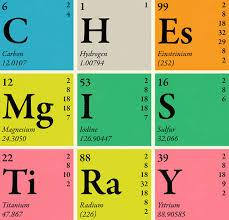# Year 9 Chemistry Mid Unit Revision

25 Questions | Attempts: 259Settings.

• 1.
The positively charged particle in an atom is the:
• A.

Protons

• B.

Neutrons

• C.

Electrons

• D.

Orbitals

• 2.
The maximum numbers of electrons in the first four energy levels of an atom are:
• A.

2, 8, 8, 18

• B.

2, 4, 6, 8

• C.

2, 14, 26, 28

• D.

2, 2, 8, 12

• 3.
When an atom loses an electron, it becomes a:
• A.

Negative ion

• B.

Positive ion

• C.

Polyatomic ion

• D.

Neutral ion

• 4.
Which of the following terms could be used to describe the matter in this image?
• A.

Single atoms

• B.

Molecules

• C.

Compound

• D.

Element

• 5.
Which of the following terms could be used to describe the matter in this image?
• A.

Single atoms

• B.

Molecules

• C.

Compound

• D.

Element

• 6.
Which of the following terms could be used to describe the matter in this image?
• A.

Single atoms

• B.

Molecules

• C.

Elements

• D.

Mixture

• 7.
Which of the following word equations is correct for a reaction between copper and oxygen?
• A.

Copper + oxygen -> carbon dioxide

• B.

Copper + oxygen -> copper sulfide

• C.

Copper + oxygen -> copper oxide

• D.

Copper + oxygen -> copper iodide

• 8.
The chemical symbols of chlorine, potassium, copper, sodium, nitrogen and iron in order are:
• A.

Cl, P, Co, So, Ni, I

• B.

Cl, K, Cu, Na, N, Fe

• C.

Cl, K, Co, So, N, Fe

• D.

Cl, Po, Co, Na, N, Fe

• 9.
What is a chemical compound?
• A.

A combination of two protons and two electrons

• B.

A combination of two or more chemical elements bonded together

• C.

A mixture of two or more chemical elements

• D.

Any substance that contains chemically bonded particles

• 10.
Which one of the following is a mixture?
• A.

Sea water

• B.

Distilled water

• C.

Iron oxide

• D.

Oxygen

• 11.
Natural gas is mainly methane (CH4). What two elements are found in methane?
• A.

Cobolt and helium

• B.

Copper and holmium

• C.

Carbon and hydrogen

• D.

Carbon and helium

• 12.
Which of the following statements is NOT true?
• A.

There are approximately 118 identified elements

• B.

An element is defined by its number of electrons - this is how elements are ordered in the periodic table

• C.

An element's atomic number indicates its number of protons

• D.

You can find an element's neutrons by subtracting the number of protons from the atomic mass

• 13.
Cadmium's atomic number is ______________. Thus, an atom of cadmium has ___________ protons and ___________ electrons. If cadmium has 64 neutrons, its mass number is ___________.
• 14.
A beryllium atom has 4 protons, 5 neutrons, and 4 electrons. What is the mass of this atom?
• A.

5

• B.

8

• C.

9

• D.

13

• 15.
Which electronic structure is correct for an element with 17 electrons?
• A.

1,7

• B.

10, 7

• C.

2, 8, 7

• D.

2, 8, 8, 18

• 16.
What do the elements in a group have the same number of?
• A.

Electrons

• B.

Occupied shells

• C.

Protons

• D.

Valence electrons

• 17.
Which of these electronic structures belongs to a noble gas?
• A.

2

• B.

2, 7

• C.

2, 8, 2

• D.

2, 8, 7

• 18.
Following the law of conservation of mass, if a student mixes 250g of sugar, 250g of water and 5g of colouring, the product will have a mass of:
• A.

500

• B.

505

• C.

503

• D.

55

• 19.
Which of the following statements best defines the law of conservation of mass?
• A.

Mass cannot be created but it can be destroyed under extreme pressures.

• B.

When added to a system, energy can destroy mass.

• C.

The mass of a closed system cannot change over time; mass cannot be created nor destroyed.

• D.

Mass cannot be conserved during a chemical reaction; a little bit of mass is always lost.

• 20.
Why is it important to know the 'sensitivity' of your balance when doing a conservation of matter experiment?
• A.

To eliminate all measurement errors.

• B.

To change data as necessary.

• C.

To make sure you prove the law.

• D.

To know the level of accuracy of your measurements.

• 21.
In a chemical reaction, the mass of the products must always equal the mass of:
• A.

The gases released

• B.

The reactants

• C.

The solids put into the reaction

• D.

The whole system

• 22.
Which of the following correctly match the X in this atom?
• A.

It is positively charged

• B.

It is negatively charged

• C.

It is the location of protons

• D.

It is the location of electrons

• E.

It is the location of neutrons

• F.

It contains most of the mass of an atom

• G.

It contains most of the volume of an atom

• 23.
¹⁴₆C and ¹²₆C are examples of carbon:
• A.

Valences

• B.

Ions

• C.

Isotopes

• D.

Molecules

## Related TopicsBack to top
×

Wait!
Here's an interesting quiz for you.